# References

1. Problem and history

“Reference” is any sign joining a sentence with any preceding sentence. References are the means for making the text a compact entity. They are the basis for evaluating text cohesion. In qualitative linguistics there is an ample literature on different kinds of references and their behavior. The sentences joined by a commoh reference are called hrebs.

The only law, known in the literature as “Hřebíček´s reference law”, originates from Hřebíček´s (1985) derivation. Altmann (1988: 81-85) proposed merely some further problems for investigation. The law was corroborated on many Turkish texts. Formula (4) supports Herdan´s version of the type-token ratio (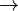$\rightarrow$).

2. Hypothesis

The number of references in text depends on the number of words and the number of sentences.

“Word” is every word-like entity (token) in the text. “Sentence” in written texts is demarcated by orthographic signs.

3. Derivation

Let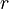$r$ = number of references in text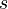$s$ = number of sentences in text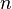$n$ = number of word tokens in text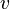$v$ = number of word types in text (vocabulary of the text)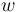$w$ = vocabulary richness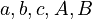$a, b, c, A, B$ = constants

Hřebíček´s assumptions:

(i) the richer the vocabulary, the smaller the number of references,

(ii) the more sentences in the text, the greater the number of references.

The change in the number of references relative to the change in the vocabulary richness is proportional to the number of sentences,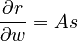$\frac{\partial r}{\partial w}=As$,

and, at the same time, the change in the number of references relative to the change in the number of sentences is proportional to the vocabulary richness of the text,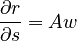$\frac{\partial r}{\partial s}=Aw$.

This yields the following solution<:

(1)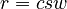$r=csw\quad$ (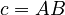$c = AB$).

Taking the simplest interpretation of vocabulary richness$w$ as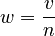$w=\frac{v}{n}$,

we obtain

(2)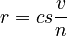$r=cs\frac{v}{n}$ .

Using Herdan´s (1966: 76) type-token ratio ($\rightarrow$) to express the vocabulary of the text as a power function of its length,

(3)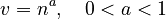$v=n^a,\quad 0 < a < 1$,

and inserting this in (2), one obtains

(4)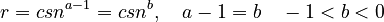$r= csn^{a-1}=csn^b, \quad a-1 = b \quad -1 < b < 0$,

which meets assumptions (i) and (ii).

Example: The course of references in a Turkish text

Hřebíček (1992) examined the course of references in several Turkish texts. One of these cases is shown in Table 1.

4. Authors: U. Strauss, G. Altmann

5. References

Altmann, G. (1988a). Wiederholungen in Texten. Bochum, Brockmeyer.

Hřebíček, L. (1985). Text as a unit and co-references. In: Ballmer, Th.T. (ed.), Linguistic dynamics: 190-198. New York, de Gruyter.

Hřebíček, L. (1986). Cohesion in Ottoman poetic texts. Archiv orientální 54,252-256.

Hřebíček, L. (1989). A syntactic variable on the text level. Glottometrika 10, 204-218.

Hřebíček, L. (1992). Text in communication: Supra-sentence structure. Bochum, Brockmeyer.

Hřebíček, L. (2000). Variation in sequences. Prague: Oriental Institute. Hřebíček 1985, 1986, 1989, 1992, 2000; Altmann 1988.

Hřebíček, L. (2006). Text laws. In: Köhler, R., Altmann, G., Piotrowski, R.G. (eds.), Quantitative Linguistics. An International Handbook: 348-361. Berlin: de Gruyter.

Mehler, A. (2006). Eigenschaften der textuellen Einheiten und Systeme. In: Köhler, R., Altmann, G., Piotrowski, R.G. (eds.), Quantitative Linguistics. An International Handbook: 325-348. Berlin: de Gruyter.

[[[Das zeichnen geht schwer, da zwei unabhängige Variablen drin sind. Mit Harvard Graphics wirds]]]